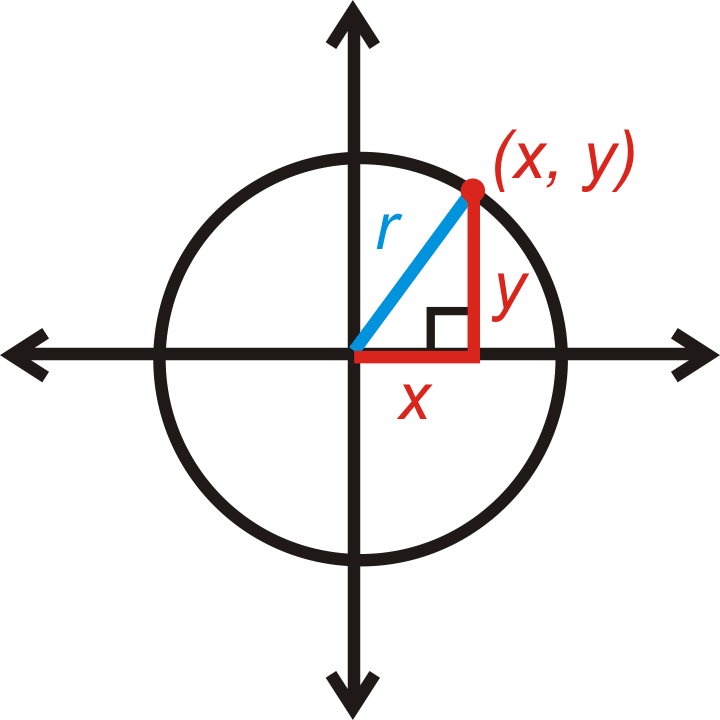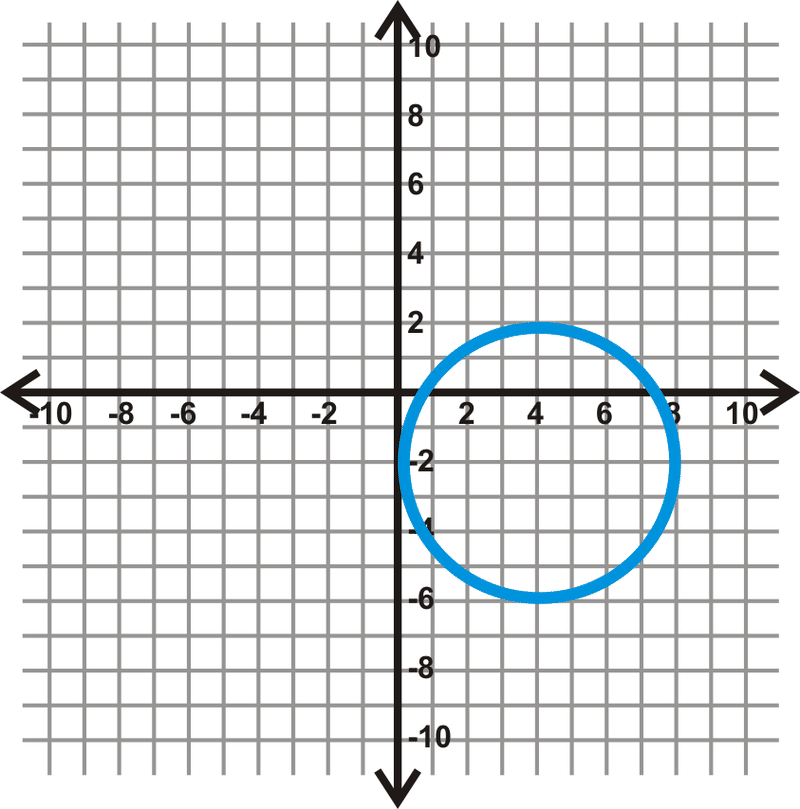# 6.21: Circles in the Coordinate Plane

•• Contributed by CK12
• CK12

Graph a circle. Use $$(h, k)$$ as the center and a point on the circle. Formula: $$(x-h)^2 + (y-k)^2 = r^2$$ where $$(h, k)$$ is the center and $$r$$ is the radius.

Recall that a circle is the set of all points in a plane that are the same distance from the center. This definition can be used to find an equation of a circle in the coordinate plane.Figure $$\PageIndex{1}$$

Let’s start with the circle centered at $$(0, 0)$$. If $$(x,y)$$ is a point on the circle, then the distance from the center to this point would be the radius, r. x is the horizontal distance and y is the vertical distance. This forms a right triangle. From the Pythagorean Theorem, the equation of a circle centered at the origin is $$x^2+y^2=r^2$$.

The center does not always have to be on $$(0, 0)$$. If it is not, then we label the center $$(h,k)$$. We would then use the Distance Formula to find the length of the radius.Figure $$\PageIndex{2}$$

$$r=\sqrt{(x−h)^2+(y−k)^2}$$

If you square both sides of this equation, then you would have the standard equation of a circle. The standard equation of a circle with center $$(h,k)$$ and radius $$r$$ is $$r^2=(x−h)^2+(y−k)^2$$.

What if you were given the length of the radius of a circle and the coordinates of its center? How could you write the equation of the circle in the coordinate plane?

Example $$\PageIndex{1}$$

Find the center and radius of the following circle.

$$(x+2)^2+(y−5)^2=49$$

Solution

Rewrite the equation as $$(x−(−2))^2+(y−5)^2=7^2$$. The center is $$(-2, 5)$$ and $$r=7$$.

Keep in mind that, due to the minus signs in the formula, the coordinates of the center have the opposite signs of what they may initially appear to be.

Example $$\PageIndex{2}$$

Find the center and radius of the following circle.

Find the equation of the circle with center $$(4, -1)$$ and which passes through $$(-1, 2)$$.

Solution

First plug in the center to the standard equation.

\begin{aligned} (x−4)^2+(y−(−1))^2&=r^2 \\ (x−4)^2+(y+1)^2&=r^2\end{aligned}

Now, plug in (-1, 2) for $$x$$ and $$y$$ and solve for $$r$$.

\begin{aligned} (−1−4)^2+(2+1)^2=r^2 \\ (−5)^2+(3)^2&=r^2 \\ 25+9&=r^2 \\ 34&=r^2\end{aligned}

Substituting in $$34$$ for $$r^2$$, the equation is $$(x−4)^2+(y+1)^2=34$$.

Example $$\PageIndex{3}$$

Graph $$x^2+y^2=9$$.

Solution

The center is $$(0, 0)$$. Its radius is the square root of 9, or 3. Plot the center, plot the points that are 3 units to the right, left, up, and down from the center and then connect these four points to form a circle.Figure $$\PageIndex{3}$$

Example $$\PageIndex{4$$

Find the equation of the circle below.Figure $$\PageIndex{4}$$

Solution

First locate the center. Draw in the horizontal and vertical diameters to see where they intersect.Figure $$\PageIndex{5}$$

From this, we see that the center is $$(-3, 3)$$. If we count the units from the center to the circle on either of these diameters, we find $$r=6$$. Plugging this into the equation of a circle, we get: $$(x−(−3))^2+(y−3)^2=6^2$$ or $$(x+3)^2+(y−3)^2=36$$.

Example $$\PageIndex{5}$$

Determine if the following points are on $$(x+1)^2+(y−5)^2=50$$.

Solution

Plug in the points for x and y in $$(x+1)^2+(y−5)^2=50$$.

1. $$(8, -3)$$

\begin{aligned} (8+1)^2+(−3−5)^2&=50 \\ 9^2+(−8)^2&=50 \\ 81+64 &\neq 50\end{aligned}

$$(8, -3)$$ is not on the circle

1. $$(-2, -2)$$

\begin{aligned} (−2+1)^2+(−2−5)^2&=50 \\ (−1)^2+(−7)^2&=50 \\ 1+49&=50\end{aligned}

$$(-2, -2)$$ is on the circle

## Review

Find the center and radius of each circle. Then, graph each circle.

1. $$(x+5)^2+(y−3)^2=16$$
2. $$x^2+(y+8)^2=4 3. \((x−7)^2+(y−10)^2=20 4. \((x+2)^2+y^2=8 Find the equation of the circles below. 1.Figure \(\PageIndex{6}$$
2.Figure $$\PageIndex{7}$$
3.Figure $$\PageIndex{8}$$
4.Figure $$\PageIndex{9}$$
5. Is (-7, 3) on $$(x+1)^2+(y−6)^2=45$$?
6. Is (9, -1) on $$(x−2)^2+(y−2)^2=60$$?
7. Is (-4, -3) on $$(x+3)^2+(y−3)^2=37$$?
8. Is (5, -3) on $$(x+1)^2+(y−6)^2=45$$?

Find the equation of the circle with the given center and point on the circle.

1. center: (2, 3), point: (-4, -1)
2. center: (10, 0), point: (5, 2)
3. center: (-3, 8), point: (7, -2)
4. center: (6, -6), point: (-9, 4)

## Vocabulary

Term Definition
circle The set of all points that are the same distance away from a specific point, called the center.
radius The distance from the center to the outer rim of a circle.
Distance Formula The distance between two points $$(x_1, y_1)$$ and $$(x_2, y_2)$$ can be defined as $$d=\sqrt{(x_2−x_1)^2+(y_2−y_1)^2}$$.
Origin The origin is the point of intersection of the x and y axes on the Cartesian plane. The coordinates of the origin are (0, 0).

Interactive Element

Video: Graphing Circles Principles - Basic

Activities: Circles in the Coordinate Plane Discussion Questions

Study Aids: Properties of a Circle Study Guide

Practice: Circles in the Coordinate Plane

Real World: GPS Geometry